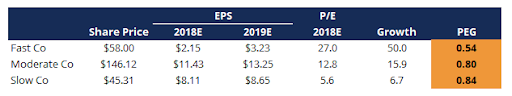1. What is the PEG Ratio?
2. PEG Ratio vs P/E Ratio: What’s the Difference?
3. PEG Ratio Example
4. (Projected or Actual) Earnings Growth
5. The Bottom Line: PEG Ratio

Everybody wants to know which stock to buy and which stock to sell. But how do you distinguish between the two?

For fundamental investors, it’s based on value.

Which stocks are trading below their true value — and should be bought — and which stocks are trading above their true value and should be sold?

The concept is simple, but the execution is difficult because of one thing:

How do we determine value?

For most, the P/E ratio is the answer. Despite its broad acceptance, the P/E leaves out one of the most important factors in investing: growth.

The PEG ratio corrects that error.

## What is the PEG Ratio?

The price/earnings-to-growth ratio (PEG ratio), is a metric that investors use to value a stock by comparing its current market price to its earnings, and to its expected future growth.

The PEG ratio was invented because a company that is expected to grow its earnings, all else being equal, is worth more than a company with little growth opportunity.

This fact is why growth companies have higher P/E ratios than value companies — investors are willing to pay more for higher potential growth.

Earnings growth (or lack thereof) and how much you pay for that growth is what matters when making investments into equities.

A low P/E ratio may make a stock look attractive, but understanding why its P/E is so low is what matters. More often than not, low P/E’s are the result of low growth prospects.

Adding a company’s expected future growth into the equation allows you to adjust companies with higher P/E ratios that also have higher growth rates, and compare them to companies with lower P/E ratios and lower growth rates.

There are two primary drawbacks for using the PEG ratio, both having to do with calculating the estimated growth:

1. The EPS growth rate is based on forward-looking expectations and (like the weather) will not be 100% correct. It will also be less and less right the further into the future you look.
2. The growth rate typically only considers a short time period, about 1-3 years. While this may give a fuller picture of a company’s value than the P/E, it may overstate the benefit of buying a stock with only a couple of years of high growth.

Each of these has the power to significantly skew a stock’s attractiveness. Take care when estimating future earnings (or trusting sources that attempt to do so).

### PEG Ratio Formula

The PEG ratio formula is as follows:

PEG = (Share Price / EPS) / EPS Growth Rate

The price-to-earnings ratio (P/E ratio) serves as the numerator in the equation, and is then divided by the expected earnings-per-share growth rate.

## PEG Ratio vs P/E Ratio: What’s the Difference?

The PEG ratio enhances the P/E ratio by factoring in expected earnings into the calculation.

By factoring in expected earnings growth, the PEG ratio presents a more complete picture of whether a stock is over or undervalued.

## PEG Ratio ExampleTo illustrate, consider the following table:

• To calculate the P/E ratio for Moderate Co, divide its current price of \$146.12 by its 2018 EPS of \$11.43 = 12.8x
• To calculate its EPS growth rate, take the estimated 2019 EPS of \$13.25 and subtract the actual 2018 EPS of \$11.43, then divide by \$11.43 = 15.9%
• To calculate the PEG ratio, divide its P/E ratio of 12.8x by its expected growth of 15.9% = 0.80

Many investors would avoid Fast Co like the plague because of its high P/E ratio, asking themselves, “How can somebody pay 27x earnings!?”

But this misses the point.

Fast Co trades at a high P/E ratio because of its expected earnings growth.

When we divide all 3 companies by their expected growth rates, Fast Co now looks the cheapest, given its increasing EPS.

## (Projected or Actual) Earnings Growth

As mentioned earlier, there is considerable risk in using estimated earnings to drive the valuation process.

As with any metric, the accuracy of the PEG ratio depends on the accuracy of the inputs.

Actual earnings can vary widely from expectations. Because of this, while it is a helpful adjustment to the P/E, the PEG ratio can still only be one of the various factors when making investment decisions.

## The Bottom Line: PEG Ratio

The PEG ratio is a more meaningful measure of value than the P/E ratio alone. By taking potential growth into consideration, the PEG ratio allows you to evaluate a company's current share price in relation to its future earnings.

However, the quality of the results from the formula depends on the quality of the inputs.

Future growth must be estimated, there’s no way around that. And with estimates come errors.

Thus, the PEG ratio should be considered a useful tool for evaluating equities and should be seen as simply one more input to guide and inform investment decisions.